Math Courses / Course / Chapter

# What is the Standard Error of the Estimate? - Formula & Examples

Lesson Transcript
Instructor
Betsy Chesnutt

Betsy has a Ph.D. in biomedical engineering from the University of Memphis, M.S. from the University of Virginia, and B.S. from Mississippi State University. She has over 10 years of experience developing STEM curriculum and teaching physics, engineering, and biology.

Expert Contributor
Kathryn Boddie

Kathryn has taught high school or university mathematics for over 10 years. She has a Ph.D. in Applied Mathematics from the University of Wisconsin-Milwaukee, an M.S. in Mathematics from Florida State University, and a B.S. in Mathematics from the University of Wisconsin-Madison.

The standard error of the estimate is used in statistics to show closely the sample mean matches the population mean. Study the definition of standard error, the formula used to calculate it through examples, and how to interpret standard error. Updated: 12/23/2021

## Definition of Standard Error

As part of a high school project, let's say you decide to measure how tall each of the players on your school's basketball team are. You find that the average height of the players on the team is 72 inches. Is this a good estimate of the height of all basketball players? How would you know, and is there a way to quantify exactly HOW good of an estimate this measure is? In fact, there's a way to quantify this, but before you can answer these questions, we first need to think about the difference between a sample and a population.

In statistics, the word sample refers to the specific group of data collected. In this case, the sample would be the data you collected on the height of players on your school's team. A population is the entire group from which the sample was drawn. This could include all high school basketball players, all basketball players at any level, or any other group. There are many ways to define a population, and you always need to be very clear about what your population is. For this project, let's assume that you want to compare the heights of the basketball players on your school's team to the heights of all high school basketball players. Therefore, the population would be all high school basketball players.

Now, in order to determine how well your sample represents the population, do you need to go out and measure how tall every single high school basketball player is? No, of course you couldn't do this! Instead, you can calculate the standard error, which tells you how well your sample mean estimates the true population mean. A large standard error would mean that there is a lot of variability in the population, so different samples would give you different mean values. A small standard error would mean that the population is more uniform, so your sample mean is likely to be close to the population mean.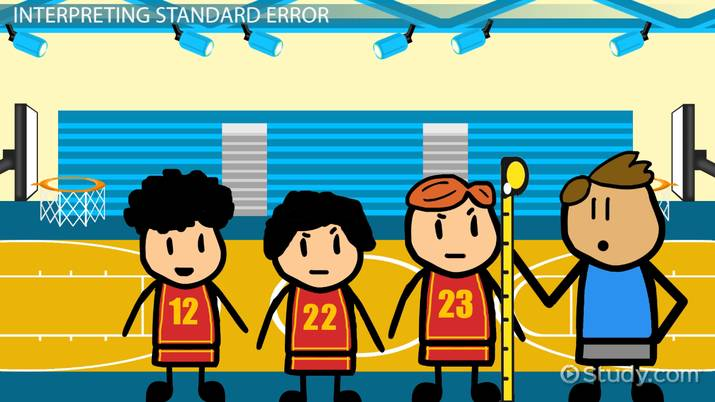An error occurred trying to load this video.

Try refreshing the page, or contact customer support.

Coming up next: What is Random Sampling? - Definition, Conditions & Measures

### You're on a roll. Keep up the good work!

Replay
Your next lesson will play in 10 seconds
• 0:00 Definition of Standard Error
• 1:55 Calculating a Standard Error
• 3:00 Example of Standard Error
• 4:17 Interpreting Standard Error
• 5:51 Lesson Summary
Save Timeline
Autoplay
Autoplay
Speed Speed

## Calculating a Standard Error

To calculate the standard error, follow these steps:

1. Record the number of measurements (n) and calculate the sample mean (μ). This is just the average of all the measurements.
2. Calculate how much each measurement deviates from the mean (subtract the sample mean from the measurement).
3. Square all the deviations calculated in step 2 and add these together:
Σ(xi - μ)²
4. Divide the sum from step 3 by one less than the total number of measurements (n - 1).
5. Take the square root of the number you got in step 4. This is known as the standard deviation (σ).
6. Finally, divide the standard deviation from step 5 by the square root of the number of measurements (n) to get the standard error of your estimate.

You'll often see these steps expressed as formulas like these, where σ is the standard deviation and SE is the standard error: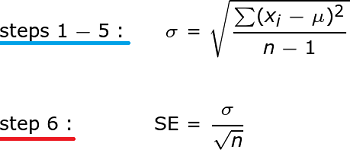## Example of Standard Error

There are a lot of steps required to find the standard error. Let's continue with our high school basketball player height example to make sure you understand how to perform these calculations.

Let's assume that this was the data you collected on basketball player height at your school: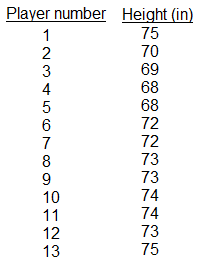The first step to find the standard error is to find the sample mean. You would do that by adding up all the heights and then dividing by the total number of measurements (n = 13). This would give you a sample mean of 72.

Next, calculate the difference between the sample mean and each measurement, square all these values, and then add them all up. This is easier to do if you make a table like the one you are looking at on your screen: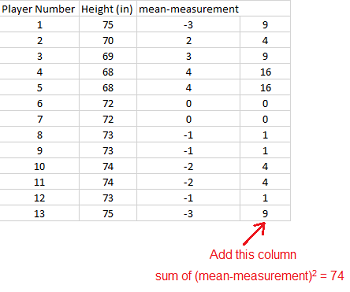Then, divide the sum you just calculated by n - 1 and take the square root to get the standard deviation.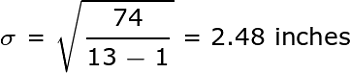Finally, to calculate the standard error of your estimate, divide the standard deviation by the square root of the number of measurements (remember: n = 13). So the standard error equals: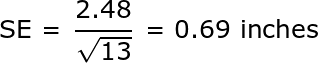So there you go.

To unlock this lesson you must be a Study.com Member.

## Practice Problems

Finding the standard error for data sets can be daunting - there are a lot of steps in the formulas needed. However, with practice, it gets easier. In the following examples, students will calculate the standard error of the estimate for two different data sets and find the 95% confidence interval for those data sets. After completing all examples, students will feel more confident finding the standard error and working with multi-step formulas.

#### Problem 1

Find the standard error of the estimate of the mean weight of high school football players using the data given of weights of high school football players from your school. Then find a 95% confidence interval for the data.

Player Number Weight in Pounds
1 150
2 203
3 176
4 190
5 168
6 193
7 189
8 178
9 197
10 172

#### Problem 2

Find the standard error of the estimate for the average number of children in a household in your city by using the data collected from a sample of households in your city. Then find a 95% confidence interval for the data.

Household Number of Children
1 2
2 3
3 1
4 0
5 5
6 2
7 1
8 4

## Solutions

#### Solution to Problem 1

First, find the mean for the data set of 10 players. The mean is the sum of the weights divided by 10 and equals 181.6 pounds. We will create a table to evaluate the sum in the formula.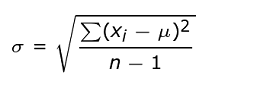Player Number Weight in Pounds Mean - Measurement (Mean - Measurement)^2
1 150 -31.6 998.56
2 203 21.4 457.96
3 176 -5.6 31.36
4 190 8.4 70.56
5 168 -13.6 184.96
6 193 11.4 129.96
7 189 7.4 54.76
8 178 -3.6 12.96
9 197 15.4 237.16
10 172 -9.6 92.16

Adding the last column in the table gives 2270.4. Then using the formula with n = 10, we have: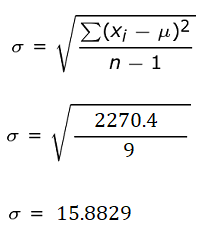Then to get the standard error, we divide this result by the square root of n (with n = 10) to get a standard error of 5.0226 pounds.

To get the 95% confidence interval, we add and subtract 1.96 * 5.0226 from our sample mean to get a 95% confidence interval of 171.7557 to 191.4443 pounds.

#### Solution to Problem 2

Start by finding the sample mean of number of children in the household: 2.25 children. Then create a table to find the sum part of the formula:Household Number of Children measurement - mean (measurement - mean)^2
1 2 -0.25 0.0625
2 3 0.75 0.5625
3 1 -1.25 1.5625
4 0 -2.25 5.0625
5 5 2.75 7.5625
6 2 -0.25 0.0625
7 1 -1.25 1.5625
8 4 1.75 3.0625

Adding the last column, we get 19.5. Using this in the formula with n = 8, we have: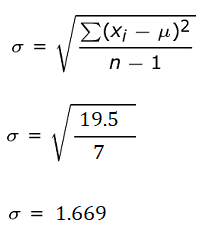To find the standard error, we just divide by the square root of n (with n = 8) to get the standard error of 0.59 children.

To find a 95% confidence interval, add and subtract 1.96 * 0.59 to the sample mean to get 1.0936 to 3.4064 children.

### Register to view this lesson

Are you a student or a teacher?

Back

### Resources created by teachers for teachers

Over 30,000 video lessons & teaching resources‐all in one place.Video lessonsQuizzes & WorksheetsClassroom IntegrationLesson Plans

I would definitely recommend Study.com to my colleagues. It’s like a teacher waved a magic wand and did the work for me. I feel like it’s a lifeline.

Jennifer B.
TeacherCreate an account to start this course today
Used by over 30 million students worldwide